﻿

### On the semifield planes of order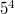$5^4$ and dimension 2 over the kernel

#### Abstract

Enter section title between curly braces In this article we consider the problem of determining all non-Desarguesian semifield planes of order$5^4$ and kernel GF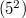$(5^2)$. We show that the class of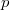$p$-primitive planes is the largest class and besides those the only other semifield planes in the class under study are the generalized twisted field planes. We conjecture that in general these two classes include all the non-Desarguesian semifield planes of order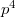$p^4$ and kernel GF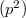$(p^2)$.

DOI Code: 10.1285/i15900932v22n1p75

Keywords: Semifields; Semifields planes; Finite affine planes

Full Text: PDF